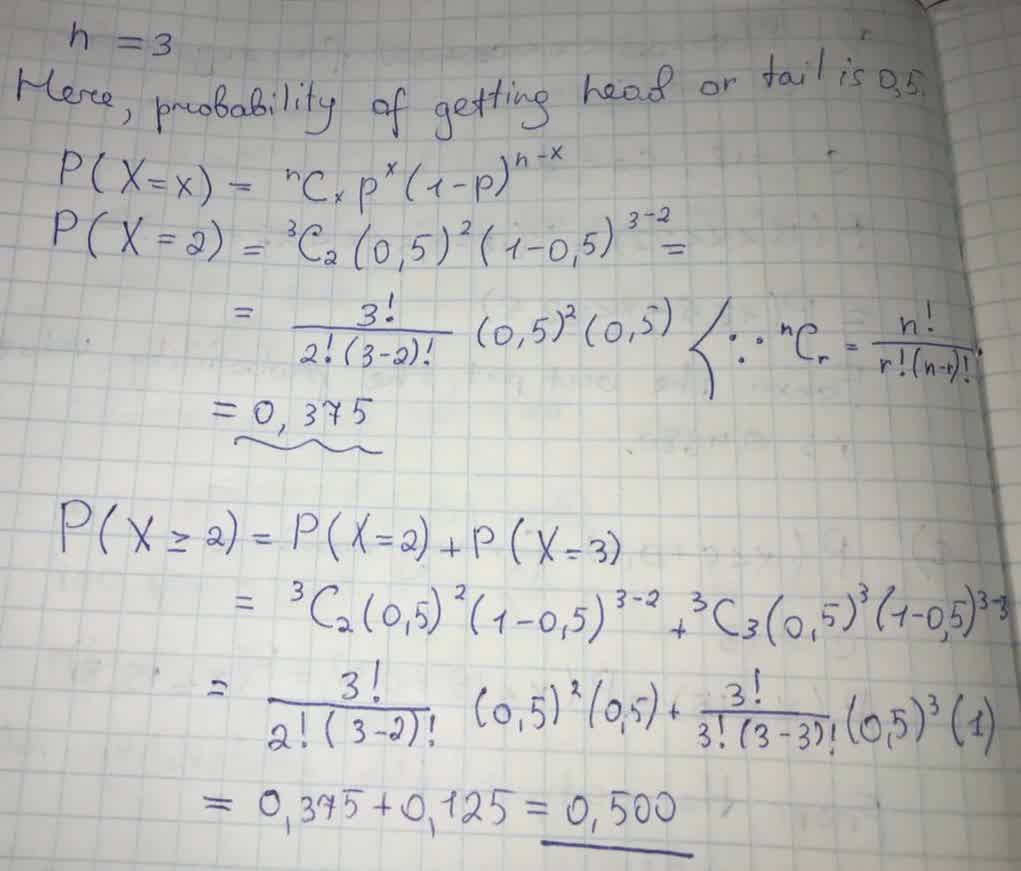# Next, look up the probability in the binomial probability distribution table. (b) Find the probability of getting exactly two heads.Ramsey 2021-09-02 Answered
A fair quarter is flipped three times.
For each of the following probabilities, use the formula for the binomial distribution and a calculator to compute the requested probability.
Next, look up the probability in the binomial probability distribution table. (Enter your answers to three decimal places.)
(b) Find the probability of getting exactly two heads.
(c) Find the probability of getting two or more heads.
You can still ask an expert for help

• Questions are typically answered in as fast as 30 minutes

Solve your problem for the price of one coffee

• Math expert for every subject
• Pay only if we can solve itpivonie8
Explanation is given below